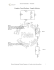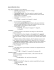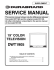# Sample ELEC 311 Final Questions

## Transcription

Sample ELEC 311 Final Questions
```Sample ELEC 311 Final Questions
Diode AC small-signal analysis
1. Determine the bias voltage, VBIAS in the circuit shown in Fig. 1. When vin = 100 sin(2π1000t) mV, the
total output voltage, vO(t) is as shown in Fig. 2. (10 marks)
Figure 1
Figure 2
Diode DC analysis
R = 1 kΩ
R=1 kΩ
2. Determine the unknown quantities below. Assume that the diodes are ideal, and please show that any
assumptions you make regarding the states of the diodes are valid. (10 marks)
V1 = ___________. V2 = ___________.
Zener Diode Analysis
3. Consider the circuit shown below in Fig 3. V+ = 12 V +/- 1V. The Zener diode has a VZ0= 5 V and rz
= 10 Ω. For this question, you can assume that this model is valid for any non-zero reverse breakdown
current (IZ > 0). That is, IZK = 0 mA. The resistance R has not yet been specified. There are several
quantities that can be calculated for a Zener diode based regulator. As an example, one can calculate the
maximum current that the regulator can supply while maintaining the desired output voltage. Please see
the last row in the table. The equation in the bottom right cell of the table shows an expression for this
quantity. A quick examination of this expression leads us to the conclusion that to maximize the
maximum available current, we should decrease R, as indicated in the last row of the table.
Please fill in the columns “Direction of R….” and “Rationale” for each of the remaining three
specifications.
Design Goal:
V+
IR
R
IZ
+
VL
-
Fig. 3
IL
Minimize the power
connected. I.e., P=V+*IR
Improve the line
regulation (consider the
is connected). I.e.,
minimize ΔVL/ΔV+
regulation. I.e., minimize
current IL is connected.
Increase the maximum
current that the regulator
can supply while
maintaining a DC output
voltage near 5 V.
Direction of R for
satisfying goal
(i.e., Increase R, decrease
R or R typically has little
effect on the goal)
Rationale
V + − VZO
R
Reducing R will
increase IMAX
I MAX =
Make R small
RC=10 kΩ
RC=10 kΩ
RC=10 kΩ
RB1=10 kΩ
RB1=0.8 kΩ
RC=10 kΩ
RE=8 kΩ
RB1=5 kΩ
RB1=10 kΩ
BJT DC analysis
4. Below are four possible configurations for establishing DC bias conditions for a BJT. Rank the four
of them from best to worst in terms of the collector current’s insensitivity to β, temperature and the
device’s IS. That is, the best circuit is the one whose collector current will change the least if β,
temperature or the device’s saturation current (IS) changes (2-4 marks?)
Best circuit: ___________.
Second best:____________.
Two worst circuits (in no particular order): _____ & ______.
5. Assuming β=100, for Circuit A, determine the BJT’s DC terminal voltages and currents. Please label
the terminal voltages and currents on the schematic. (10 marks)
RD=10 kΩ
MOSFET Analysis
6. This question deals with the circuits in Figure 4, below. The purpose of this question is to analyze an
amplifier that contains a biasing scheme that is frequently used in integrated circuit applications. For this
• For MOSFETs M1 and M2, assume:
o Vt = 0.5 V
o µnCOX(W/L) = 200 µA/V2.
o λ = 0 V-1
• IREF = 100 µA
Figure 4
a) Please find VB and ID in the section of the circuit on the left.
b) If we connect the node labeled “A” to the node labeled “VB” will the node voltage VB change? If
yes, what is its new value? If not, why does it not change? (Hint: consider how much current will
flow into terminal A).
c) Now, with the two circuits connected, analyze the circuit to determine the DC bias conditions for
M2 and the DC output voltage VO.
d) Find the AC small-signal input resistance (RIN) for the node VB for the circuit in the left half of
Fig. 4.
e) Draw the complete AC small-signal equivalent circuit for the circuits in Fig 4 when VB is
connected to A. You should represent the left half of the circuit by the resistance RIN that you
have calculated.
f) Find the AC small-signal voltage gain vo/vsig.
```

### Electronics_exercises_files/extra 2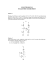### INDUCTOR An inductor, also called a coil or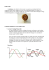### File### www.chlorofil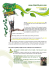### Vincent Camus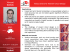### KIRCHOFF`S VOLTAGE LAW: EXAMPLE 1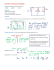### Lab Work #2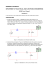### The Series RLC Circuit: Group Worksheet Intro Part 1: Resistive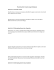### Why try Circuit?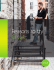### LIGHT EMITTING DIODE CHARACTERISTICS (SAMPLE LAB WRITEUP) Be sure the title block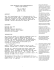### Meter Main with Load Center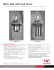### CMGT 235 Quiz #8 Show all calculations! Name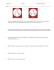### Electronics EECE2412 — Spring 2016 Exam #1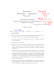### Alexander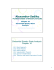### 12BH7 TJ FullMusic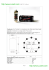### paper - ap pgecet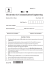### 6SN7 SE TJ FullMusic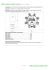### Common Emitter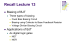### Electronics II. 3. measurement : Tuned circuits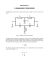### Complex Circuit Redraws - Sample Solution